### Linear elastic materials for large strains (Ciarlet model)

In  it is explained that substituting the infinitesimal strains in the classical Hooke law by the Lagrangian strain and the stress by the Piola-Kirchoff stress of the second kind does not lead to a physically sensible material law. In particular, such a model (also called St-Venant-Kirchoff material) does not exhibit large stresses when compressing the volume of the material to nearly zero. An alternative for isotropic materials is the following stored-energy function developed by Ciarlet  (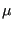and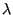are Lamé's constants):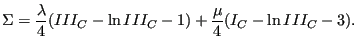(295)

The stress-strain relation amounts to (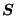is the Piola-Kirchoff stress of the second kind) :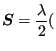det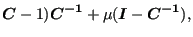(296)

and the derivative ofwith respect to the Green tensor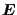reads (component notation):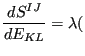det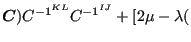det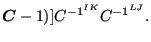(297)

This model was implemented into CalculiX by Sven Kaßbohm. The definition consists of a *MATERIAL card defining the name of the material. This name HAS TO START WITH ”CIARLET_EL” but can be up to 80 characters long. Thus, the last 70 characters can be freely chosen by the user. Within the material definition a *USER MATERIAL card has to be used satisfying:

First line:

• *USER MATERIAL
• Enter the CONSTANTS parameter and its value, i.e. 2.

Following line:

•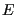(Young's modulus).
•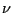(Poisson's coefficient).
• Temperature.

Repeat this line if needed to define complete temperature dependence.

For this model, there are no internal state variables.

Example:

*MATERIAL,NAME=CIARLET_EL
*USER MATERIAL,CONSTANTS=2
210000.,.3,400.


defines an isotropic material with elastic constants=210000. and=0.3 for a temperature of 400 (units appropriately chosen by the user). Recall that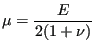(298)

and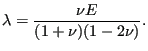(299)